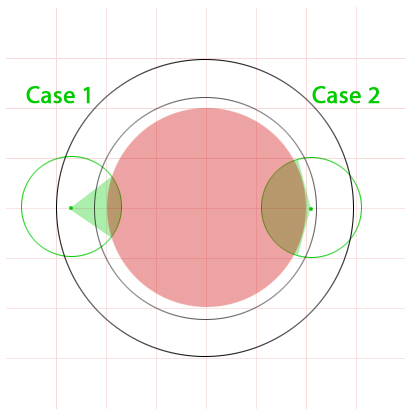BrainDen.com - Brain Teasers
• 0

# Probability of missing the circle

## Question

Since nobody was brave enough to attack my 'bum' question, here is one that sounds more 'mathy'...

Suppose you have a box with a square base area of 16 square inches.  There is a circle drawn inside the base which shares the same center point.  The diameter of this circle is 2 inches.  Lastly, assume that you have a line segment with length of 1 inch which will randomly appear within the base of the box.  What is the probability that this line segment will not intersect the circle?

## Recommended Posts

• 0

Spoiler

The box and circle are shown in red. Consider the line to be contained in a 1” diameter green circle that shares its center. If the line center is outside the gray circle of diameter sqrt (5) ` 2.236, (circles A, B and E) the probability of intersection is given by twice the fraction of green arc inside the red circle. (Twice because either end can intersect the circle.) In the gray area or on a 3” circle, (circles A and E) it’s zero. In the white area inside the gray circle (circle D) the probability is slightly larger than the arc length in the red circle. On or in the red circle (not shown), it’s certain to intersect. The gray area is ~0.558 of the area of the square, so the probability of not intersecting is at least that much. By the above reasoning, it’s more.Spoiler

We made an assumption whose validity is uncertain owing the OP not definitively saying how the line “randomly appears.” This has assumed the center of the line could appear anywhere in the box, with random orientation. When the line center is within 1” of the box edge, not all orientations are possible (if the line it to be totally contained by the box), and the probability therefore should decrease, becoming zero for the line center to appear at the box’s edge. That calculation is more like a problem on a math exam than the answer to a puzzle, so I assume instead that the distribution of the line center is uniform everywhere inside the box. We can then assume the edge of the box modifies the orientation as needed.

SpoilerWhen the line center appears in the white area, there are two cases. In Case 1 (outside the sqrt(5) circle) the fraction of arc length inside the red circle can be calculated directly as a function of the distance d from the center of the box: Cos (theta) = (d2-3)/2d. The probability of not intersecting is then (pi-theta)/pi. In case 2 (inside the sqrt(5) circle) the angle is measured between tangent lines, so that it approaches pi when d=2. You know what? It's feeling a bit more like math than logic at this point Someone else is welcome to do it.

Anyway, the answer comes from adding the gray area probability (.558) to the appropriate integrals for non-intersecting as a function of d from 3 to sqrt(5) and from sqrt(5) to 2.

That solves the puzzle in principle.

## Join the conversation

You can post now and register later. If you have an account, sign in now to post with your account.×   Pasted as rich text.   Paste as plain text instead

Only 75 emoji are allowed.

×   Your previous content has been restored.   Clear editor

×   You cannot paste images directly. Upload or insert images from URL.